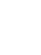# Alan Modelinde Çarpma İşlemiİndirGöm(Embed) Kapat Bu benzetimin çalışır kopyasını göm Bu benzetimin çalışır halini eklemek için bu HTML'i kullan.Genişlik ve boyunu HTML kısmından değiştirebilirsiniz Tıklandığında benzetim başlatacak bir görüntü iliştir
Çalıştırmak için tıklayın
Ekranda görüntülemek için bu HTML kodunu kullanın "Çalıştır için tıklayın". Partial Products Factors Çarpma PhET ekibini destekleyenlerSizin gibi eğitimciler.

### İçerik

• Partial Products
• Factors
• Çarpma
• Area Model

### Description

Build rectangles of various sizes and relate multiplication to area. Discover new strategies for multiplying large numbers. Use the game screen to test your problem solving strategies!

### Örnek Öğrenim Hedefleri

• Recognize that area represents the product of two numbers and is additive.
• Represent a multiplication problem as the area of a rectangle, proportionally or using generic area.
• Develop and justify a strategy to determine the product of two multi-digit numbers by representing the product as an area or the sum of areas.

### Standards Alignment

#### Common Core - Math

3.MD.C.7
Relate area to the operations of multiplication and addition.
3.MD.C.7c
Use tiling to show in a concrete case that the area of a rectangle with whole-number side lengths a and b + c is the sum of a × b and a × c. Use area models to represent the distributive property in mathematical reasoning.
3.MD.C.7d
Recognize area as additive. Find areas of rectilinear figures by decomposing them into non-overlapping rectangles and adding the areas of the non-overlapping parts, applying this technique to solve real world problems.
4.NBT.B.5
Multiply a whole number of up to four digits by a one-digit whole number, and multiply two two-digit numbers, using strategies based on place value and the properties of operations. Illustrate and explain the calculation by using equations, rectangular arrays, and/or area models.
6.EE.A.3
Apply the properties of operations to generate equivalent expressions. For example, apply the distributive property to the expression 3 (2 + x) to produce the equivalent expression 6 + 3x; apply the distributive property to the expression 24x + 18y to produce the equivalent expression 6 (4x + 3y); apply properties of operations to y + y + y to produce the equivalent expression 3y.
6.EE.A.4
Identify when two expressions are equivalent (i.e., when the two expressions name the same number regardless of which value is substituted into them). For example, the expressions y + y + y and 3y are equivalent because they name the same number regardless of which number y stands for..
6.NS.B.4
Find the greatest common factor of two whole numbers less than or equal to 100 and the least common multiple of two whole numbers less than or equal to 12. Use the distributive property to express a sum of two whole numbers 1-100 with a common factor as a multiple of a sum of two whole numbers with no common factor. For example, express 36 + 8 as 4 (9 + 2)..
7.EE.A.1
Apply properties of operations as strategies to add, subtract, factor, and expand linear expressions with rational coefficients.
7.NS.A.2c
Apply properties of operations as strategies to multiply and divide rational numbers.
Version 1.2.1

### Teacher TipsÖğretmen el kitabı (pdf) Phet ekibi tarafından oluşturulan ipuçları içerir. ( PDF ).

### Teacher-Submitted Activities

Middle School Math Sim AlignmentAmanda McGarry Ortaokul Diğer Matematik
SECUNDARIA: Alineación PhET con programas de la SEP México (2011 y 2017)Diana López Lİse
Ortaokul
Diğer Matematik
Biyoloji
Fizik
Kimya
PREPARATORIA: Alineación de PhET con programas de la DGB México (2017)Diana López Lisans-Giriş
Lİse
Diğer Matematik
Kimya
Fizik
PRIMARIA: Alineación con programas de la SEP México (2011 y 2017)Diana López K-5
Ortaokul
Laboratuvar
Discuss
Guided
Ödev
Gösteri
Astronomi
Matematik
Kimya
Fizik
Dil Downloador RunTips
Almanca All DeutschFlächenmodell Multiplikation
Arapça All العربيةضرب مساحة نموذج
Arnavutça All shqipModeli i Syprinës: Shumëzimi
Baskça All EuskaraAzalera-modeloan biderkaketa
Boşnakça All BosanskiMatematički model površina - Množenje
Brezilya Portekizcesi All português (Brasil)Modelo de Área: Multiplicação
Çince (Geleneksel) All 中文 (台灣)Area Model Multiplication_面積模型與乘法
Çince (Sadeleştirilmiş) All 中文 (中国)面积模型乘法
Danca All DanskArealmodel, gange
Farsça All فارسیضرب مدل مساحت
Fince All suomiPinta-alamallilla kertominen
Flemenkçe All NederlandsOppervlakte model vermenigvuldiging
Fransızca All françaisModélisation de la multiplication par les aires
Gujarati All Gujaratiક્ષેત્રફળનું ગુણાકારનું મોડેલ - નિકુંજ સવાણી દ્વારા
Hintçe All हिंदीनमूने का क्षेत्रफल - गुणन
İngilizce All EnglishArea Model Multiplication
İspanyolca All españolModelo de Áreas: Multiplicaciónİspanyolca (Meksika) All español (México)Modelo de Áreas: Multiplicaciónİspanyolca (Peru) All español (Perú)Modelo de Área con multiplicación
İsveçce All svenskaAreamodellen - Multiplikation
İtalyanca All italianoRappresentazione grafica della moltiplicazione
Japonca All 日本語面積を求める
Kazakça All KazakhКөбейту жолымен ауданын есептеу
Korece All 한국어면적 모형: 곱셈
Lehçe All polskiModel pola - mnożenie
Letonca All LatviešuLauka modeļa reizinājums
Macarca All magyarTerületmodell: szorzás
Moğolca All Монгол (Монгол)Талбайн Загварын Үржвэр
Portekizce All portuguêsModelo de Área: Multiplicação
Rusça All русскийВычисление площади путем умножения
Seylanca All Sinhaleseවර්ගඵල ආකෘති ගුණ කිරීම
Sırpça All СрпскиМатематика преко површина-Множење
Ukraynaca All українськаПлощинна модель множення
Vietnamca All Tiếng ViệtPhép nhân với mô hình diện tích
Yunanca All ΕλληνικάΥπολογισμός εμβαδού - Πολλαπλασιασμός

HTML5 sims can run on iPads and Chromebooks, as well as PC, Mac, and Linux systems.

iOS 12+ Safari

Android:
Not officially supported. If you are using the HTML5 sims on Android, we recommend using the latest version of Google Chrome.

Chromebook:
The HTML5 and Flash PhET sims are supported on all Chromebooks.
Chromebook compatible sims

Windows Systems:

Macintosh Systems:

Linux Systems:

Tasarım Ekibi Harici Kütüphaneler Sayesinde
• Jonathan Olson (developer)
• Karina Hensberry
• Susan Miller
• Ariel Paul
• Kathy Perkins
• Mariah Hermsmeyer (artwork)
• Diana López Tavares (artwork)
• almond-0.2.9.js
• base64-js-1.2.0.js
• FileSaver-b8054a2.js
• font-awesome-4.5.0
• game-up-camera-1.0.0.js
• he-1.1.1.js
• himalaya-0.2.7.js
• jama-1.0.2
• jquery-2.1.0.js
• lodash-4.17.4.js
• pegjs-0.7.0.js
• seedrandom-2.4.2.js
• text-2.0.12.js
• TextEncoderLite-3c9f6f0.js